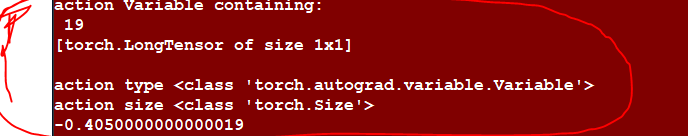# Epsilon Greedy random pick (Datastructure)

Hello there, I get the following errror when i try to convert use the .data function when apply epsilon greedy but i do not understand this because i use the same data structure for my softmax which can be seen in the output picture.

error:

``````random type <class 'torch.autograd.variable.Variable'>
-0.245000000000002
random Variable containing:
3
[torch.LongTensor of size 1x1]

-0.5
Traceback (most recent call last):
File "main.py", line 67, in <module>
training.update(currentState) #,currentState,currentState,currentState)
File "C:\Users\koch\Documents\Skole\Uni\Kandidat\4 Semester\svn_kandidat\python\Qnetwork_LSTM_20a\training.py", line 36, in update
self.action = self.brain.update(last_reward, last_signal)
File "C:\Users\koch\Documents\Skole\Uni\Kandidat\4 Semester\svn_kandidat\python\Qnetwork_LSTM_20a\DRL_Qnetwork_LSTM.py", line 175, in update
action = self.select_action(new_state)
File "C:\Users\koch\Documents\Skole\Uni\Kandidat\4 Semester\svn_kandidat\python\Qnetwork_LSTM_20a\DRL_Qnetwork_LSTM.py", line 151, in select_action
return action.data[0,0]
File "C:\Users\koch\Anaconda3\envs\py36_pytorch_kivy\lib\site-packages\torch\tensor.py", line 395, in data
raise RuntimeError('cannot call .data on a torch.Tensor: did you intend to use autograd.Variable?')
RuntimeError: cannot call .data on a torch.Tensor: did you intend to use autograd.Variable?
``````

I have the function with epsilon greedy and soft max:

``````    def select_action(self, state):
#LSTM
if self.steps_done is not 0:  # The hx,cx from the previous iteration
self.cx = Variable(self.cx.data)
self.hx = Variable(self.hx.data)

q_values, (self.hx, self.cx) = self.model((Variable(state), (self.hx, self.cx)), False)

if self.params.action_selector == 1: #Softmax
probs = F.softmax((q_values)*self.params.tau,dim=1)
#create a random draw from the probability distribution created from softmax
action = probs.multinomial()
print('action',action)
print('action type',type(action))
print('action size',type(action.size()))
elif self.params.action_selector == 2: #Epsilon Greedy
sample = random.random()
eps_threshold = self.params.eps_end + (self.params.eps_start - self.params.eps_end) * \
math.exp(-1. * self.steps_done / self.params.eps_decay)
if sample > eps_threshold:
action =  q_values.type(torch.FloatTensor).data.max(1).view(1, 1)
else:
hej = Variable(torch.LongTensor([[random.randrange(self.params.action_size+1)]]))
print('random',hej)
print('random type',type(hej))
action = hej
self.steps_done += 1
return action.data[0,0]
``````

Output when using softmax function: